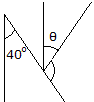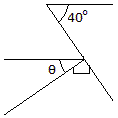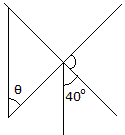# Engineering Mechanics - General Principles - Discussion

### Discussion :: General Principles - General Questions (Q.No.8)

8.Determine the angle:

 [A].= 30° [B].= 40° [C].= 60° [D].= 50°

Explanation:

No answer description available for this question.

 Sunny said: (Sep 29, 2011) In fig. 3 40+x=90 so x=50 and there mades z So @=50

 Mudasir Shaik said: (May 27, 2017) In the fig 2 [right side]. 90 + θ +40 = 180. We get the angle 40 from "the angle made by the line intersecting two parallel lines is same" --> θ = 50.